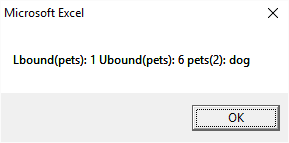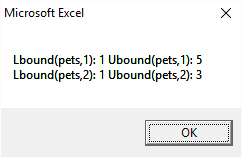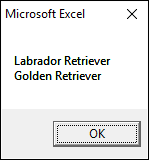Author: Oscar Cronquist Article last updated on January 16, 2018

This post will teach you how to work with excel arrays in visual basic for applications (VBA).

Why do you need arrays? Excel is much quicker executing tasks with arrays than working with values on a worksheet.

Arrays exists in your internal computer RAM memory while workbooks exists on your hard drive.

Working with data from your internal computer RAM memory is much faster.

### Declare an array variable

A variable can store one value, an array variable can store multiple values.
The following line declares pets as a String array variable. The parentheses shows that this is an array variable.

```Sub Macro1()
Dim pets() As String
End Sub
```

You can also specify how many values you want to store in the array. The macro below declares variable pets as a string array, it can store 6 values. 0 to 5.

```Sub Macro1()
Dim pets(5) As String
End Sub
```

If you want to start with 1 instead of 0 you can do that too. This variable can store 5 values, 1 to 5.

```Sub Macro1()
Dim pets(1 to 5) As String
End Sub
```

### Redimensioning an array variable

You can also change the amount of values an array variable can store any time in the macro, using the ReDim statement.

```Sub Macro1()
Dim pets(1 to 2) As String

pets(1) = "dog"
Msgbox Ubound(pets)

ReDim pets(1 to 3)

Msgbox pets(1) &amp; " " &amp; Ubound(pets)

End Macro
```

Note that this clears all previous saved array values.

### n-dimensional arrays

The example arrays shown above have one dimension but it is possible to have up to 60000 dimensions. You are probably going to use one or two dimensions most of the time.

1-dimensional arrays
You can assign values to an array in your macro or user defined function. The following macro shows you how.

```Sub Macro1()
Dim pets(1 to 2) As String
pets(1) = "dog"
pets(2) = "cat"
End Sub
```

In this macro 2 values can be stored pets(1 to 2). The index number in the pets variable tells excel where to save the value in the array. pets(index)

You can also grab values from a worksheet and save them to an array.

```Sub Macro1()
Dim pets(1 to 2) As String

pets(1) = Worksheets("Sheet1").Range("A1").value
pets(2) = Worksheets("Sheet1").Range("A2").value
End Sub
```

If you have many values this macro might do the work but this method is relatively slow.

```Sub Macro1()
Dim pets(1 to 100) As String
For i = 1 to 100
pets(i) = Worksheets("Sheet1").Range("A" &amp; i).value
Next i
End Sub
```

There is a quicker way to transfer values from a worksheet to an array that I will tell you about later in this post.

2-dimensional arrays
This macro shows you how to populate a 2 dimensional array. The first argument is the row number and the second is the column number, pets(row,column)

```Sub Macro1()
Dim pets(1 to 2, 1 to 2)

pets(1,1) = "dog"
pets(1,2) = 5
pets(2,1) = "cat"
pets(2,2) = 9
End Sub
```

This picture is a visual presentation of the array variable. The italic numbers show the position of each value in the array.3-dimensional arrays
It is also possible to have arrays with three dimensions. The best analogy I can think of is if the first argument is the row number, the second argument is column number, the third argument is then like worksheets.

```Sub Macro1()
Dim pets(1 to 2, 1 to 2, 1 to 2)

pets(1,1,1) = "dog"
pets(1,2,1) = 5
pets(2,1,1) = "cat"
pets(2,2,1) = 9
pets(1,1,2) = "snake"
pets(1,2,2) = 3
pets(2,1,2) = "fish"
pets(2,2,2) = 4
End Sub
```

### Array size

Each element or value has a index number indicating its position in the array. Arrays always have a lower and upper limit, the Lbound statement returns the lower limit and the Ubound statement returns the upper limit. These functions are very useful. If the lower limit is 1 and the upper limit is 5, the array contains 5 values.

1-dimensional array

The following macro uses the LBound and Ubound statements to determine the size of the array variable.

```Sub Macro1()
Dim pets(1 To 10)
Msgbox "LBound: " &amp; LBound(pets) &amp; " Ubound: " &amp; Ubound(pets)
End sub
```

This macro shows you how to figure out the size of a 2 dimensional array variable.

```Sub Macro1()
Dim pets(1 To 5, 1 To 3)
MsgBox "Lower limit 1-dim: " &amp; LBound(pets, 1) &amp; " Upper limit 1-dim:" &amp; UBound(pets, 1) &amp; " Lower limit 2-dim: " &amp; LBound(pets, 2) &amp; " Upper limit 2-dim: " &amp; UBound(pets, 2)
End Sub

```
```Sub Macro1()
Dim pets(1 To 5, 1 To 3, 1 To 2)
MsgBox "Lower limit 1-dim: " &amp; LBound(pets, 1) &amp; " Upper limit 1-dim:" &amp; UBound(pets, 1) &amp; " Lower limit 2-dim: " &amp; LBound(pets, 2) &amp; " Upper limit 2-dim: " &amp; UBound(pets, 2) &amp; " Lower limit 3-dim: " &amp; LBound(pets, 3) &amp; " Upper limit 3-dim: " &amp; UBound(pets, 3)
End Sub
```

### Manipulate an array

You can change dimensions of an array and still keep all values. But you need to declare the array variable with empty parentheses or you will get this compile error: "Array already dimensioned"

```Sub Macro1()
Dim pets() As String
ReDim pets(1 To 5)
pets(2) = "dog"
ReDim Preserve pets(1 To UBound(pets) + 1)
MsgBox "Lbound(pets): " &amp; LBound(pets) &amp; " Ubound(pets): " &amp; UBound(pets) &amp; "pets(2): " &amp; pets(2)
End Sub
```You can only ReDim the last dimension, the following array has 2 dimensions, the boundaries are 1 to 5 and 1 to 2.

```Sub Macro1()
Dim pets() As String
ReDim pets(1 To 5, 1 To 2)
ReDim Preserve pets(1 to Ubound(pets,1) , 1 To UBound(pets,2) + 1)
MsgBox "Lbound(pets,1): " &amp; LBound(pets,1) &amp; " Ubound(pets,1): " &amp; UBound(pets,1) &amp; vbNewLine &amp; "Lbound(pets,2): " &amp; LBound(pets,2) &amp; " Ubound(pets,2): " &amp; UBound(pets,2)
End Sub
```

The ReDim Preserve statement changes the last dimension the 2-dimensional array in the macro above. The boundaries are now 1 to 5 and 1 to 3, this picture shows you that.Excel is really slow when it comes to manipulating arrays, it is better to try to minimize the number of times you use ReDim Preserve by guessing how many elements you need.

### Clear an array

You can use the ReDim statement to clear all saved array values.

```Sub Macro1()
Dim pets(1 to 2) As String

pets(1) = "dog"
Msgbox Ubound(pets)

ReDim pets(1 to 3)

Msgbox pets(1) &amp; " " &amp; Ubound(pets)

End Macro
```

You can also use the Erase function

```Sub Macro1()

Dim pets(1 to 2) As String

pets(1) = "cat"

Erase pets

End Sub

```

### Transfer array values to a worksheet

Macro

This macro returns an array to worksheet Sheet1

```Sub Macro1()
Dim pets(1 to 2) As String

pets(1) = "dog"
pets(2) = "cat"

Worksheets("Sheet1").Range("A1:B1") = pets

End Macro
```

As you can see it returns theses values horizontally. It is possible to return values vertically also, se Transpose later in this post.

User defined function

The following user defined function (udf) returns array values but you need to enter it as an array formula.

```Function ArrTest
Dim pets(1 to 2) As String

pets(1) = "dog"
pets(2) = "cat"

ArrTest = pets

End Macro
```

Here are the steps on how to enter an array formula:

1. Select cell A1:B1
2. Type =ArrTest()
3. Press and hold CTRL + SHIFT
4. Press Enter

If you enter the udf correctly the formula bar shows {=ArrTest()}

If you fail to enter this udf as an array formula it might show the first value in the array in all cells, the formula bar shows =ArrTest()

### Transpose an array

A 1-dimensional array is always shown horizontally on worksheet, to change that you can use the Transpose method and rearrange the values vertically.

```Sub Macro1()
Dim pets(1 to 2) As String

pets(1) = "dog"
pets(2) = "cat"

Worksheets("Sheet1").Range("A1:A2") = Application.Transpose(pets)

End Sub
```

If your array is larger than 65536 the transpose statement will return an error, this macro will not work.

```Sub Macro1()
Dim pets(1 To 65537) As String
Dim i As Single
For i = 1 To 65537
pets(i) = i
Next i
Worksheets("Sheet1").Range("A1:A65537") = Application.Transpose(pets)
End Sub
```

The Transpose statement returns Run-time error '13': Type mismatch.

However, there is a workaround to overcome this array limit. The following macro returns an array vertically to cell range A1:A65537 and Transpose is not even used. The downside is that you can't change the size of the first dimension using ReDim Preserve.

```Sub Macro1()
Dim pets(1 To 65537, 1 To 1) As Single

For i = 1 To 65537
pets(i, 1) = i
Next i

Worksheets("Sheet1").Range("A1:A65537") = pets

End Sub

```

Be aware that if you use Transpose with an array that is larger than 65536 in excel 2013 or 2016 no error is shown.

### Fetch data from a worksheet

This macro retrieves 65537 values from cell range A1:A65537 and it does it super fast.

```Sub Macro1()
Dim pets() As Variant

pets = Worksheets("Sheet1").Range("A1:A65537").Value

MsgBox "LBound(pets,1):" &amp; LBound(pets, 1) &amp; " Ubound(pets,1): " &amp; UBound(pets, 1) &amp; vbNewLine &amp; "LBound(pets,2): " &amp; LBound(pets, 2) &amp; " UBound(pets,2): " &amp; UBound(pets, 2)

End Sub

```

It returns a 2-dimensional array even if you only grab one column of data.Copy data from an excel defined table

You can also copy values from an excel defined table to an array.

```Sub Macro1()
Dim pets() As Variant

pets = Range("Table1").Value

MsgBox "LBound(pets,1):" &amp; LBound(pets, 1) &amp; " Ubound(pets,1): " &amp; UBound(pets, 1) &amp; vbNewLine &amp; "LBound(pets,2): " &amp; LBound(pets, 2) &amp; " UBound(pets,2): " &amp; UBound(pets, 2)

End Sub

```

Range("Table1").Value returns all values except the headers, use Table1[#All] if you want all values. If you want to copy only column "Name" to an array use Table1[Name].

### Copy an array

To copy an entire array simply use the equal sign.

```Sub Macro1()
Dim pets(1 To 2) As Variant
Dim dogs() As Variant

pets(1) = "Labrador Retriever"
pets(2) = "Golden Retriever"

dogs = pets

MsgBox dogs(1) &amp; vbNewLine &amp; dogs(2)
End Sub

```Copy a column to a new array

Application.Index statement allows you to copy a section of an array.

dogs = Application.Index(pets, 0, 1) copies column 1 from array pets to a new array named dogs.

Copying a column to a new array creates a 2-dimensional array but if you copy a row the new array is 1-dimensional, see the two next macro examples below.

```Sub Macro1()
Dim pets(1 To 2, 1 To 2) As Variant
Dim dogs() As Variant

pets(1, 1) = "Labrador Retriever"
pets(2, 1) = "Golden Retriever"
pets(1, 2) = "Boxer"
pets(2, 2) = "Beagle"
dogs = Application.Index(pets, 0, 1)

MsgBox dogs(1, 1) &amp; vbNewLine &amp; dogs(2, 1)
End Sub

```

Copying a row (horizontal values) from an array creates a new array with only one dimension.

```Sub Macro1()
Dim pets(1 To 2, 1 To 2) As Variant
Dim dogs() As Variant

pets(1, 1) = "Labrador Retriever"
pets(2, 1) = "Golden Retriever"
pets(1, 2) = "Boxer"
pets(2, 2) = "Beagle"
dogs = Application.Index(pets, 2, 0)

MsgBox dogs(1) &amp; vbNewLine &amp; dogs(2)

End Sub

```

As you might have suspected there is a problem with the INDEX function. It can't handle arrays larger than 65536 values and Microsoft seems to do nothing about it, the error has been there since excel 2007.

### Send an array to a macro

Macro2 creates and populates a new array and then executes Macro1 using the array as an argument.

```Sub Macro1(val() As String)

MsgBox val(1) &amp; vbNewLine &amp; val(2)
End Sub

```
```Sub Macro2()
Dim pets(1 To 2) As String
pets(1) = "Labrador Retriever"
pets(2) = "Golden Retriever"
Call Macro1(pets)
End Sub
```

### Pass an array to a function

The following two functions demonstrates how to pass arrays between functions. User defined function Func2 copies values from a worksheet to an array, it then passes the array to Func1. Func1 transposes the values and sends them back. Func2 then returns the values to a worksheet.

```Function Func1(val() As Variant)
Func1 = Application.Transpose(val)
End Function

```
```Function Func2(rng As Range)
Dim temp() As Variant
Dim temp1() As Variant

temp = rng.Value
Func2 = Func1(temp)
End Function

```

You can also send an array from a macro to a udf.

### Determine the number of dimensions in an array

User defined function CountDim counts the number of dimensions in an array.

```Function CountDim(val() As Variant)
Dim i As Single

On Error Resume Next
For i = 1 To 60000
chk = LBound(val, i)
If Err &amp;lt;&amp;gt; 0 Then Exit For
Next i
On Error GoTo 0
CountDim = i - 1
End Function

```
```Function Test()
Dim arr(1 To 10, 1 To 5, 1 To 3, 1 To 5, 1 To 3)
Test = CountDim(arr)
End Function

```

Function Test returns 5, there are five dimensions in this array:

### Split a text string

The split function allows you to separate a text string using a delimiter. It returns a 1-dimensional array (0 to n) and that makes it interesting in this context.

```Function CountWords(rng As Range)
Text = Split(rng, " ")
CountWords = UBound(Text) + 1
End Function

```